# Posters About Numbers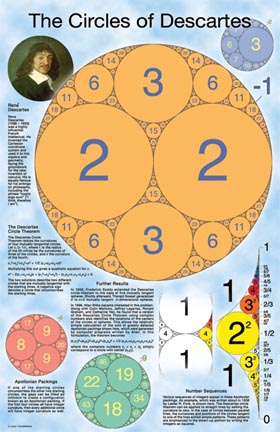The Circles of Descartes poster \$11.95 This poster describes the Descartes Circle Theorem and shows some of the beautiful and complex figures, known as Apollonian packings, that can be created using the theorem. In the early part of the 17th century, Ren Descartes described a simple relationship between the curvatures of four mutually tangent circles. Given the curvatures of three of the circles, it is possible to calculate the curvature of the fourth by a straightforward solution to a quadratic equation. (It may be the coolest thing you'll ever see done with a quadratic equation!) This allows the construction of fascinating figures know as Apollonian packings, in which an infinite number of circles fit inside a large outer circle. In fact, there are many Apollonian packings for which every circle has integer curvature. Some arrangements lead to intriguing number sequences, such as the squares (1, 4, 9, 16, 25 ...). Grades 7-12. 22" x 34".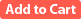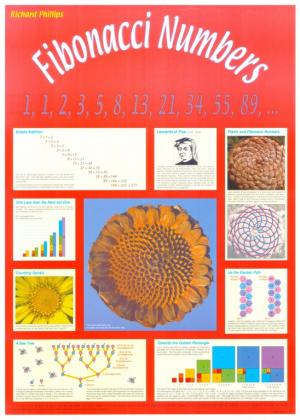Fibonacci Numbers Poster \$9.95 This poster illustrates the concept, history and natural occurrences of the Fibonacci sequence, including its role in pine cones, seed heads, and in generations of bees. 23" x 33".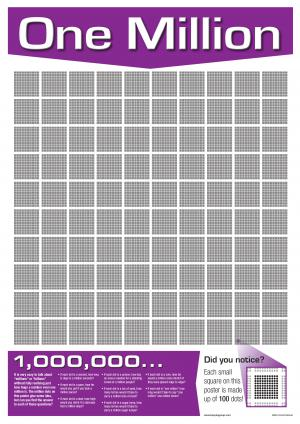One Million Poster \$9.95 This poster helps students understand what a large number like 1,000,000 really means. 10 by 10 dots form a dot for another 10 by 10 which forms an overall 10 by 10 grid. 100 dots becomes 10000 becomes 1,000,000. 23" x 33".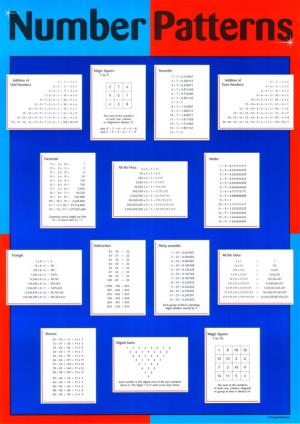Number Patterns Poster \$9.95 Explores the fascinating world of number patterns including the Addition of Odd Numbers, Magic Squares, Sevenths, the Addition of Even Numbers, Factorials, Fives, Ninths, Triangle, Subtraction, Thirty Sevenths, Ones, Elevens, Digital Sums. 23" x 33".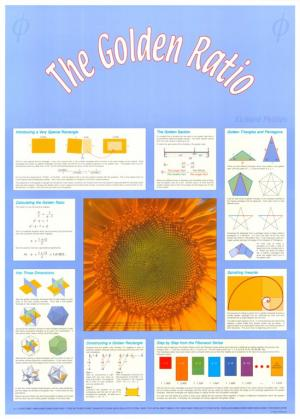The Golden Ratio Poster \$9.95 Describes the many properties of one of the most fascinating numbers. 23" x 33".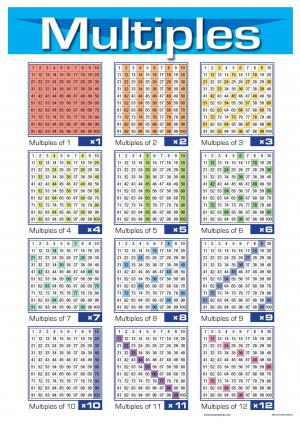Multiples Poster \$9.95 The multiples of 1 to 12 are highlighted on hundred squares, revealing the patterns that the multiples form. 23" x 33".